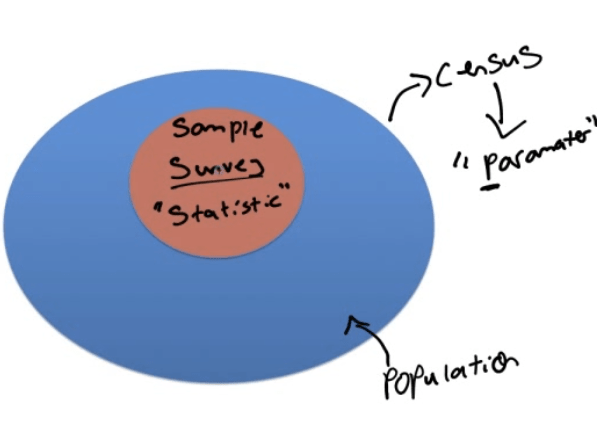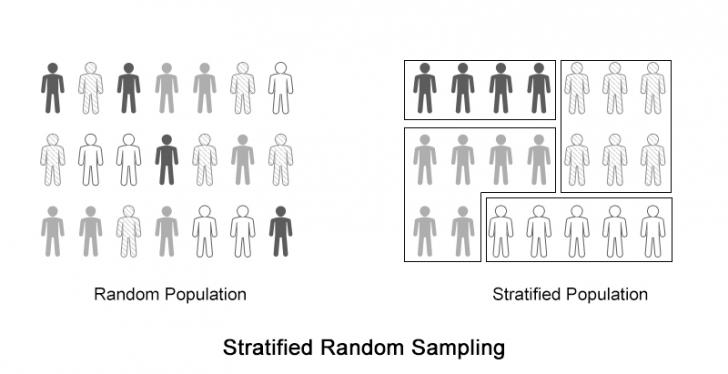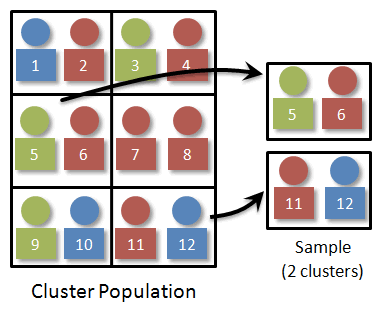# Census vs. Survey

• A parameter is a numerical description of a population characteristic

• A statistic is a numerical description of a sample characteristicCensus Population Parameter
Survey Sample Statistic

# Characteristics of a Well-Designed and Well-Conducted Survey

• Representative sample

• Must represent the population that you want to draw inference about
• Random sample

• Does not introduce bias

# Bias

• What is it?• How might it occur?

• Wording of the question

• Sample technique

• Under-coverage: miss a certain group of people

• Non-response: someone in your sample choose not to respond

# Random Sampling

• Simple Random Sample (SRS)

• Random digit table

• Calculator

• MATH ➡️ PRB ➡️ randInt(lower, upper, number of numbers)

![П-84 Pius Stver Editj(n ф TEns [NSTRUMENTS ](./media/image77.png)

• Stratified Random Sampling• Cluster Sample• Systematic Random Sample• Comparison• Example

1. If you wanted to select a sample of school children and you did this by selecting an SRS of classrooms this would be an example of what type of sampling?

• Cluster
2. If you wanted to select a sample of school children and you did this by randomly selecting 5 children from each grade this would be an example of what type of sampling?

• Stratified

# Non-Random Sampling

• Convenience Sample

• Selecting those that are easy of convenient
• Voluntary Response Sample